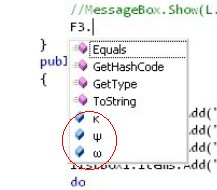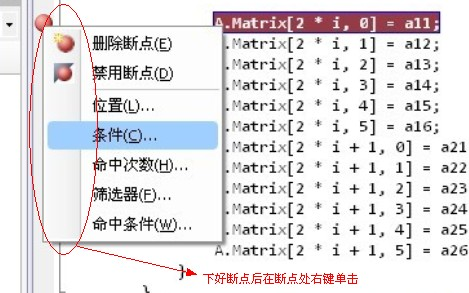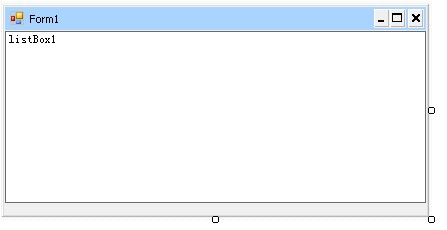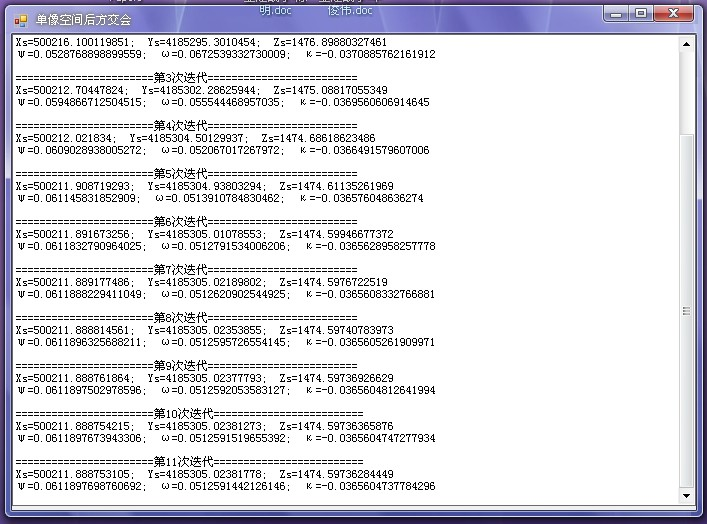# 写给同学的编程建议(续)```   public struct KZPoint   //地面控制点坐标
{
public double x;
public double y;
public double z;
}
public struct YXPoint //影像点坐标
{
public double x;
public double y;
}
public struct IData //三个输入不方便的角度
{
public double ψ;
public double ω;
public double κ;
}
```

```        double m, f, x0, y0, Xs, Ys, Zs;//m,f,x0,y0为内方位元素
int PointNum;                   //控制点的个数
double LowLimit;                //精度控制
double a1, a2, a3, b1, b2, b3, c1, c2, c3; //R矩阵各值
KZPoint[] KZPs;                   //用户输入的已知控制点
YXPoint[] YXPs;                   //用户输入的已知像点
IData F3;                         //三个输入不方便的角度
NNMatrix A, X, L;                 //平差矩阵, X=[dXs,dYs,dZs,dψ,dω,dκ]T; A[2n,6], L[2n,1]
string splitStr = @"\s{1,}";            //正则表达式的分隔符
```

```        //===========================从文本文件中读取初始数据,flilename为文本文件名=====================
public void DataFromText(string filename)
{
string text = "";                              //text变量用来临时保存我们读的每一行数据
int rows = 0;                                  //rows变量表示该文本共有多少非空行
while ((text = sr.ReadLine()) != null)
{
if (text.Trim() != "")                     //Trim方法用来截取两边的空格，如果为空行，截取后就为空了
{
rows++;                                //如果该行不为空，那么rows就加1
}
}
KZPs = new KZPoint[rows];                      //有多少非空行就有多少个点，声明点坐标数组
YXPs = new YXPoint[rows];
PointNum = rows;                               //PointNum为点的个数，声明在全局变量里面
sr.Close();
for (int i = 0; i < rows; i++)                 //用for循环来一行一行地读取数据
{
while (text == "")                         //如果这行为空就一直向下读直到读到不为空的行，也就是忽视空行
{
}
Regex reg = new Regex(splitStr);           //C#里面的正则表达式
//以空格为分隔符把这一行文本分成几个部分(我们这里是六个部分)并保存到数组中
string[] list = reg.Split(text);
KZPs[i].x = Convert.ToDouble(list);     //实现赋值
KZPs[i].y = Convert.ToDouble(list);
KZPs[i].z = Convert.ToDouble(list);
YXPs[i].x = Convert.ToDouble(list) / 1000;   //注意要将单位mm化成m
YXPs[i].y = Convert.ToDouble(list) / 1000;
}
sr.Close();
sr.Dispose();                                 //释放文件
}
```

```        //===========================初始化数据=========================================
public void InitData()
{
double tempx = 0;
double tempy = 0;
F3.κ = 0;                          //三个角度初始值都设为0
F3.ψ = 0;
F3.ω = 0;
for (int i = 0; i < PointNum; i++)
{
tempx = tempx + KZPs[i].x;
tempy = tempy + KZPs[i].y;
}
Zs = m * f;                        //按照教材上面的方法给的初值
Xs = tempx / PointNum;             //平均数作为初值
Ys = tempy / PointNum;
}
```

```        public void Calc()
{
int times = 0; //迭代的次数
//在LostBox里面显示每次运算的情况
listBox1.Items.Add("Xs=" + Xs.ToString() + "; Ys=" + Ys.ToString() + "; Zs=" + Zs.ToString());
listBox1.Items.Add("ψ=" + F3.ψ.ToString() + "; ω=" + F3.ω.ToString() + "; κ=" + F3.κ.ToString());
do
{
CalcR();//计算R矩阵
CalcL();//计算L矩阵
CalcA();//计算A矩阵
CalcX();//计算X矩阵
//算出X矩阵后我们把改正数加到参数里面去，作为下一次迭代的初始值
Xs = Xs + X.Matrix[0, 0]; Ys = Ys + X.Matrix[1, 0]; Zs = Zs + X.Matrix[2, 0];
F3.ψ = F3.ψ + X.Matrix[3, 0]; F3.ω = F3.ω + X.Matrix[4, 0]; F3.κ = F3.κ + X.Matrix[5, 0];
//迭代次数加1
times = times + 1; //迭代次数加1
//在LostBox里面显示每次运算的情况
} while ((Math.Abs(X.Matrix[0, 0]) > LowLimit) || (Math.Abs(X.Matrix[1, 0]) > LowLimit) || (Math.Abs(X.Matrix[2, 0]) > LowLimit) || (Math.Abs(X.Matrix[3, 0]) > LowLimit) || (Math.Abs(X.Matrix[4, 0]) > LowLimit) || (Math.Abs(X.Matrix[5, 0]) > LowLimit));
//这个do...while循环实现迭代，其中由while条件来判断什么时候结束迭代，其中LowLimit是我们设置的精度，定义在全局变量中
}
```

```    //===========================计算R矩阵==========================================
public void CalcR()//用到的是教材32页公式3-9
{
a1 = Math.Cos(F3.ψ) * Math.Cos(F3.κ) - Math.Sin(F3.ψ) * Math.Sin(F3.ω) * Math.Sin(F3.κ);
a2 = -Math.Cos(F3.ψ) * Math.Sin(F3.κ) - Math.Sin(F3.ψ) * Math.Sin(F3.ω) * Math.Cos(F3.κ);
a3 = -Math.Sin(F3.ψ) * Math.Cos(F3.ω);
b1 = Math.Cos(F3.ω) * Math.Sin(F3.κ);
b2 = Math.Cos(F3.ω) * Math.Cos(F3.κ);
b3 = -Math.Sin(F3.ω);
c1 = Math.Sin(F3.ψ) * Math.Cos(F3.κ) + Math.Cos(F3.ψ) * Math.Sin(F3.ω) * Math.Sin(F3.κ);
c2 = -Math.Sin(F3.ψ) * Math.Sin(F3.κ) + Math.Cos(F3.ψ) * Math.Sin(F3.ω) * Math.Cos(F3.κ);
c3 = Math.Cos(F3.ψ) * Math.Cos(F3.ω);
}
//===========================计算L矩阵==========================================
public void CalcL()//用到的是教材62页公式5-4
{
L = new NNMatrix(2 * PointNum, 1);
double JSx = 0;
double JSy = 0;
for (int i = 0; i < PointNum; i++)
{
//算近似值用到教材34页公式3-15，其中加上了x0，y0不为0的情况
JSx = x0 / 1000 - f * ((a1 * (KZPs[i].x - Xs) + b1 * (KZPs[i].y - Ys) + c1 * (KZPs[i].z - Zs)) / (a3 * (KZPs[i].x - Xs) + b3 * (KZPs[i].y - Ys) + c3 * (KZPs[i].z - Zs)));
JSy = y0 / 1000 - f * ((a2 * (KZPs[i].x - Xs) + b2 * (KZPs[i].y - Ys) + c2 * (KZPs[i].z - Zs)) / (a3 * (KZPs[i].x - Xs) + b3 * (KZPs[i].y - Ys) + c3 * (KZPs[i].z - Zs)));
//给L矩阵赋值
L.Matrix[2 * i, 0] = YXPs[i].x - JSx;
L.Matrix[2 * i + 1, 0] = YXPs[i].y - JSy;
}
}
//===========================计算A矩阵==========================================
public void CalcA()//用到教材63页公式5-8及66页公式5-9b
{
double a11, a12, a13, a21, a22, a23, a14, a15, a16, a24, a25, a26, Z1;
A = new NNMatrix(2 * PointNum, 6);
for (int i = 0; i < PointNum; i++)
{
Z1 = a3 * (KZPs[i].x - Xs) + b3 * (KZPs[i].y - Ys) + c3 * (KZPs[i].z - Zs);
a11 = (a1 * f + a3 * YXPs[i].x) / Z1;
a12 = (b1 * f + b3 * YXPs[i].x) / Z1;
a13 = (c1 * f + c3 * YXPs[i].x) / Z1;
a21 = (a2 * f + a3 * YXPs[i].y) / Z1;
a22 = (b2 * f + b3 * YXPs[i].y) / Z1;
a23 = (c2 * f + c3 * YXPs[i].y) / Z1;
a14 = YXPs[i].y * Math.Sin(F3.ω) - ((YXPs[i].x * (YXPs[i].x * Math.Cos(F3.κ) - YXPs[i].y * Math.Sin(F3.κ))) / f + f * Math.Cos(F3.κ)) * Math.Cos(F3.ω);
a15 = -f * Math.Sin(F3.κ) - YXPs[i].x * (YXPs[i].x * Math.Sin(F3.κ) + YXPs[i].y * Math.Cos(F3.κ)) / f;
a16 = YXPs[i].y;
a24 = -YXPs[i].x * Math.Sin(F3.ω) - ((YXPs[i].x * (YXPs[i].x * Math.Cos(F3.κ) - YXPs[i].y * Math.Sin(F3.κ))) / f - f * Math.Sin(F3.κ)) * Math.Cos(F3.ω);
a25 = -f * Math.Cos(F3.κ) - YXPs[i].y * (YXPs[i].x * Math.Sin(F3.κ) + YXPs[i].y * Math.Cos(F3.κ)) / f;
a26 = -YXPs[i].x;
//给A矩阵赋值
A.Matrix[2 * i, 0] = a11;
A.Matrix[2 * i, 1] = a12;
A.Matrix[2 * i, 2] = a13;
A.Matrix[2 * i, 3] = a14;
A.Matrix[2 * i, 4] = a15;
A.Matrix[2 * i, 5] = a16;
A.Matrix[2 * i + 1, 0] = a21;
A.Matrix[2 * i + 1, 1] = a22;
A.Matrix[2 * i + 1, 2] = a23;
A.Matrix[2 * i + 1, 3] = a24;
A.Matrix[2 * i + 1, 4] = a25;
A.Matrix[2 * i + 1, 5] = a26;
}
}
//===========================计算X矩阵==========================================
public void CalcX()//用到了教材63页公式5-6，主要为矩阵运算，矩阵运算代码及用法见附录中的链接
{
NNMatrix AT = new NNMatrix(6, 2 * PointNum);//声明矩阵A的转置为6行，两倍点个数列
NNMatrix ATA = new NNMatrix(6, 6);          //ATA为A的转置乘以A
NNMatrix ATA1 = new NNMatrix(6, 6);         //ATA1为ATA的逆
NNMatrix ATA1AT = new NNMatrix(6, 2 * PointNum);  //ATA1AT为ATA1乘以A的转置
X = new NNMatrix(6, 1);
AT = NNMatrix.Transpos(A);  //求AT
ATA = AT * A;
ATA1 = NNMatrix.Invers(ATA); //求ATA的逆
ATA1AT = ATA1 * AT;
X = ATA1AT * L;
}
```

```        private void Form1_Load(object sender, EventArgs e)
{
this.Text = "单像空间后方交会";
DataFromText("data.txt"); //读取数据
//初始化参数
x0 = 0;
y0 = 0;
m = 50000;
f = 0.0281359;
LowLimit = 0.000029;
//初始化数据
InitData();
//进行迭代计算并显示结果
Calc();
}
```

OK，到这里这个程序就算是完成了。我们将数据都填好到data.txt这个文件中，然后把data.txt拷到和我们程序一个目录下，然后我们F5调试运行，效果如图：```       private void Form1_DragEnter(object sender, DragEventArgs e)
{
else e.Effect = DragDropEffects.None;
}
```

```     private void Form1_DragDrop(object sender, DragEventArgs e)
{
//MessageBox.Show(((System.Array)e.Data.GetData(DataFormats.FileDrop)).GetValue(0).ToString());
listBox1.Items.Clear();
DataFromText(((System.Array)e.Data.GetData(DataFormats.FileDrop)).GetValue(0).ToString());
InitData();
Calc();
}
```